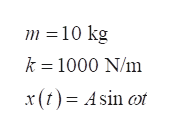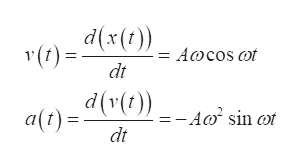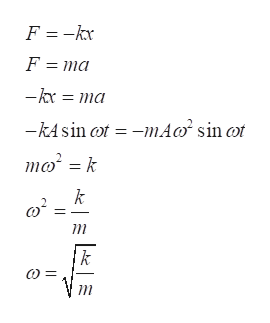A horizontal block spring oscillator of mass 10 kg on a frictionless table and spring constant k=1000 N/m is pulled 1 m to the right and released:a) Find at t=3 sec: angular frequency, frequency, period, position, velocity, acceleration, and force on blockb) Draw the acceleration function of the block over two periods

Question

A horizontal block spring oscillator of mass 10 kg on a frictionless table and spring constant k=1000 N/m is pulled 1 m to the right and released:

a) Find at t=3 sec: angular frequency, frequency, period, position, velocity, acceleration, and force on block

b) Draw the acceleration function of the block over two periods

Step 1

Let m be the mass of the block, and k be the spring constant of the spring. Assume x to be the motion described by the block as a sinusoidal function as below.help_outlineImage Transcriptionclosem 10 kg k =1000 N/m x(tAsin t fullscreen
Step 2

Write the expression for the velocity and acceleration of the mass in terms of time.help_outlineImage Transcriptionclose)x Awcos t dt a) A0 sin ot dt fullscreen
Step 3

At any interval of time, the forces on the block must be in equilibrium.

Write the expression for the equilib...help_outlineImage TranscriptioncloseF =-kx F ma -kr ma mA -kA sin ot sin ot mok k fullscreen

Want to see the full answer?

See Solution

Want to see this answer and more?

Our solutions are written by experts, many with advanced degrees, and available 24/7

See Solution
Tagged in

Vibrations